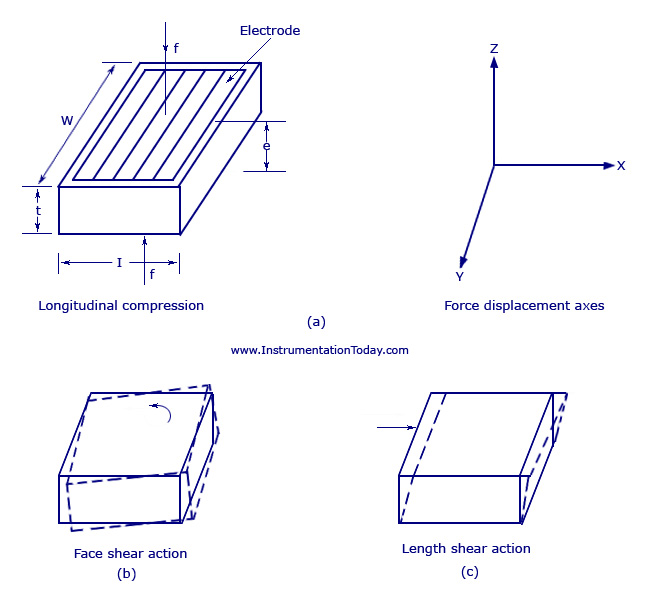UXDE dot Net Wordpress Themes

# Piezoelectric Transducer

A piezoelectric crystal transducer/sensor is an active sensor and it does not need the help of an external power as it is self-generating. It is important to know the basics of a piezoelectric quartz crystal and piezoelectric effect before going into details about the transducer.

#### Piezoelectric Quartz Crystal

A quartz crystal is a piezoelectric material that can generate a voltage proportional to the stress applied upon it. For the application, a natural quartz crystal has to be cut in the shape of a thin plate of rectangular or oval shape of uniform thickness.  Each crystal has three sets of axes – Optical axes, three electrical axes OX1, OX2, and OX3 with 120 degree with each other, and three mechanical axes OY1,OY2 and OY3 also at 120 degree with each other. The mechanical axes will be at right angles to the electrical axes. Some of the parameters that decide the nature of the crystal for the application are

• Angle at which the wafer is cut from natural quartz crystal
• Plate thickness
• Dimension of the plate
• Means of mounting

#### Piezoelectric Effect

The X-Y axis of a piezoelectric crystal and its cutting technique is shown in the figure below.

X-Y Axes of a Piezoelectric Crystal

The direction, perpendicular to the largest face, is the cut axis referred to.

If an electric stress is applied in the directions of an electric axis (X-axis), a mechanical strain is produced in the direction of the Y-axis, which is perpendicular to the relevant X-axis. Similarly, if a mechanical strain is given along the Y-axis, electrical charges will be produced on the faces of the crystal, perpendicular to the X-axis which is at right angles to the Y-axis.

Some of the materials that inherit piezo-electric effect are quartz crystal, Rochelle salt, barium titanate, and so on. The main advantages of these crystals are that they have high mechanical and thermal state capability, capability of withstanding high order of strain, low leakage, and good frequency response, and so on.

A piezoelectric transducer may be operated in one of the several modes as shown in the figure below.Piezoelectric Crystal

#### Piezoelectric Transducer

The main principle of a piezoelectric transducer is that a force, when applied on the quartz crystal, produces electric charges on the crystal surface.  The charge thus produced can be called as piezoelectricity. Piezo electricity can be defined as the electrical polarization produced by mechanical strain on certain class of crystals. The rate of charge produced will be proportional to the rate of change of force applied as input. As the charge produced is very small, a charge amplifier is needed so as to produce an output voltage big enough to be measured. The device is also known to be mechanically stiff. For example, if a force of 15 kiloN is given to the transducer, it may only deflect to a maximum of 0.002mm. But the output response may be as high as 100KiloHz.This proves that the device is best applicable for dynamic measurement.

The figure shows a conventional piezoelectric transducer with a piezoelectric crystal inserted between a solid base and the force summing member. If a force is applied on the pressure port, the same force will fall on the force summing member. Thus a potential difference will be generated on the crystal due to its property. The voltage produced will be proportional to the magnitude of the applied force.

#### Applications

1. Due to its excellent frequency response, it is normally used as an accelerometer, where the output is in the order of (1-30) mV per gravity of acceleration.
2. The device is usually designed for use as a pre-tensional bolt so that both tensional and compression force measurements can be made.
3. Can be used for measuring force, pressure and displacement in terms of voltage.

1. Very high frequency response.
2. Self generating, so no need of external source.
3. Simple to use as they have small dimensions and large measuring range.
4. Barium titanate and quartz can be made in any desired shape and form. It also has a large dielectric constant. The crystal axis is selectable by orienting the direction of orientation.

1. It is not suitable for measurement in static condition.
2. Since the device operates with the small electric charge, they need high impedance cable for electrical interface.
3. The output may vary according to the temperature variation of the crystal.
4. The relative humidity rises above 85% or falls below 35%, its output will be affected. If so, it has to be coated with wax or polymer material.1.nidhi

where can i buy this piezoelectric transducer

2.Patrick

I get that Piezoelectic crystals & ceramics produce an electrical charge but what piezoelectic compound produces the most discharge

3.Amin

yeah, could you share where can i buy these piezoelectric transducer?

i want to research some thing ASAP.
could anyone help me?

4.arifah

is it the same as piezoelectric transducer amplifier

5.vishnu

how much output voltage it will produce….???

6.yash tomar

How much electricity produce by a piezoelectric transducers

7.Bharath

How much output voltage will piezoelectric transducer produce?